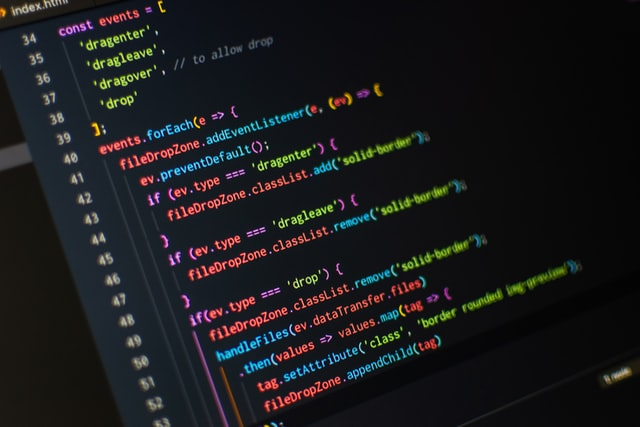# Longest common subsequence in JavaPhoto by Pankaj Patel on Unsplash

In this post we will see how to solve a problem that is to look for the longest common subsequence of two Strings that we receive as input parameters.

To solve this we will use a technique that is called dynamic programming. This technique uses the fact that a problem can be break into sub-problems in a recursive manner and using the results of previous sub-problems allow us to avoid repeating previous computations.

In this particular problem we will use a matrix called `DP` that at any given position (i, j) will have calculated the previous number characters that form a subsequence in both input Strings.

``````
public class LongestCommonSubsequence {

public int calculateLongestCommonSubsequence(String a, String b) {

int N = a.length();
int M = b.length();

if (N == 0 || M == 0) {
return 0;
}

int[][] DP = new int[N][M];

if (a.charAt(0) == b.charAt(0)) {
DP = 1;
}
for (int i = 1; i < N; i++) {
DP[i] = (DP[i - 1] == 1 || a.charAt(i) == b.charAt(0)) ? 1 : 0;
}
for (int j = 1; j < M; j++) {
DP[j] = (DP[j - 1] == 1 || a.charAt(0) == b.charAt(j)) ? 1 : 0;
}
for (int i = 1; i < N; i++) {
for (int j = 1; j < M; j++) {
DP[i][j] = Math.max(DP[i - 1][j], DP[i][j - 1]);
if (a.charAt(i) == b.charAt(j)) {
DP[i][j] = Math.max(DP[i][j], 1 + DP[i - 1][j - 1]);
}
}
}
return DP[N - 1][M - 1];
}

public static void main(String[] args) {
LongestCommonSubsequence longestCommonSubsequence = new LongestCommonSubsequence();
System.out.println(longestCommonSubsequence.calculateLongestCommonSubsequence("alex", "alexander"));
}
}

``````

### Conclusion

In this post we have seen how to solve the problem of finding the longest common subsequence between two Strings that we receive as input parameters using dynamic programming.

I won't give your address to anyone else, won't send you any spam, and you can unsubscribe at any time.
Disclaimer: Opinions are my own and not the views of my employer

Updated: